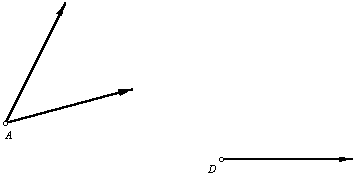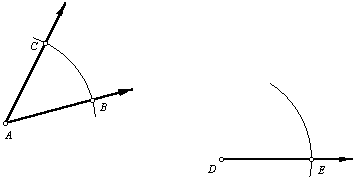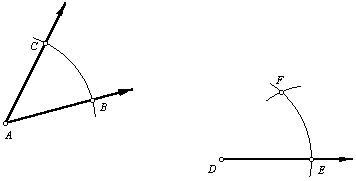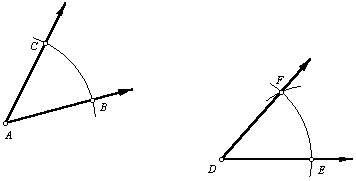Construct an angle congruent to a given angle.

 1. To draw an angle congruent to ÐA, begin by drawing a ray with endpoint D.2. Place the compass on point A and draw an arc across both sides of the angle. Without changing the compass radius, place the compass on point D and draw a long arc crossing the ray. Label the three intersection points as shown.3. Set the compass so that its radius is BC. Place the compass on point E and draw an arc intersecting the one drawn in the previous step. Label the intersection point F.4. Use the straightedge to draw ray DF. ÐEDF @ ÐBACBack to Geometry Construction Reference